## Representation of Alternating Quantity on Complex Plane Assignment Help

Assignment Help: >> Complex Representation of Alternating Quantities - Representation of Alternating Quantity on Complex Plane

Representation of Alternating Quantity on Complex Plane:

In complex plane, x-axis represents the real axis (reference axis) and y-axis represents the imaginary axis ( j-axis). Figure illustrates the representation of complex quantity on complex plane.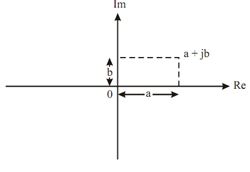Fig: complex plane

Polar Representation

Any alternating quantity may be represented in polar form (magnitude - angle form) as under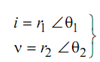Rectangular to Polar Conversion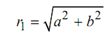and

θ1  = tan-1   (b/a)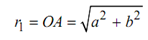Also

and

θ2  = tan-1   (d/c)

In this way, rectangular quantities a + jb and c + jd can be converted into corresponding polar form, i.e.

r1 ∠ θ1              and           r2  ∠ θ2

In Figure

and θ1 is the angle made of line OA from the horizontal axis are illustrate,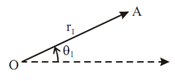Fig

Polar to Rectangular Conversion

r1 ∠ θ1 may be written as :

r1 e j θ1 ,

Then by Euler's theorem :

r1 e j θ1 = r1 [cos θ1 + j sin θ1 ]

= r1 cos θ1 + j r1 sin θ1

So,

a = r1 cos θ1

and      b = r1 sin θ1#### Assured A++ Grade

Get guaranteed satisfaction & time on delivery in every assignment order you paid with us! We ensure premium quality solution document along with free turntin report!

All rights reserved! Copyrights ©2019-2020 ExpertsMind IT Educational Pvt Ltd